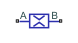# Local Resistance (IL)

Pipe resistances in an isothermal liquid system

Since R2020a

•Libraries:
Simscape / Fluids / Isothermal Liquid / Pipes & Fittings

## Description

The Local Resistance (IL) block models pressure loss due to user-defined pipe resistances in an isothermal liquid system. You can specify different loss coefficients for forward and reversed flows through the pipe segment. For pipe bends, you can also choose to use the Isothermal Liquid library blocks Pipe Bend (IL) and Elbow (IL) or, for area changes, the Area Change (IL) block.

The loss factor is parameterized by either a constant relationship based on the pipe pressure or by user-supplied tabular data for loss coefficients based on the Reynolds number.

### Constant Loss Factor

Segments with loss factors that remain constant over a range of flow velocities are calculated as:

`${k}_{loss}={k}_{loss,BA}+\frac{\left({k}_{loss,AB}-{k}_{loss,BA}\right)}{2}\left[\mathrm{tanh}\left(\frac{3\Delta p}{\Delta {p}_{crit}}\right)+1\right],$`

where:

• kloss,AB and kloss,BA are the Forward flow loss coefficient (from A to B) and Reverse flow loss coefficient (from B to A) parameters, respectively.

• Δp is the pressure difference pApB.

The critical pressure difference, Δpcrit, is the pressure differential associated with the Critical Reynolds number, Recrit, which is the flow regime transition point between laminar and turbulent flow:

`$\Delta {p}_{crit}=\frac{\overline{\rho }}{2}{k}_{loss,crit}{\left(\frac{\nu {\mathrm{Re}}_{crit}}{{D}_{h}}\right)}^{2},$`

where:

• kloss,crit is the loss factor associated with the critical pressure, and is based on an average of the forward and reverse loss coefficients.

• ν is the fluid kinematic viscosity.

• $\overline{\rho }$ is the average fluid density.

• Dh is the segment hydraulic diameter, which is the equivalent diameter of a pipe with a non-circular cross-section: ${D}_{h}=\sqrt{\frac{4}{\pi {A}_{flow}}}.$, where Aflow is the Flow area.

### Tabulated Loss Coefficient

The loss coefficient can alternatively be interpolated from user-provided Reynolds number and loss coefficient data. The vector of Reynolds numbers can have both positive and negative values, indicating forward and reverse flow, respectively: ${k}_{loss}=TLU\left(\mathrm{Re}\right).$

### Mass Flow Rate

Mass is conserved through the valve:

`${\stackrel{˙}{m}}_{A}+{\stackrel{˙}{m}}_{B}=0.$`

The mass flow rate through the valve is calculated as:

`$\stackrel{˙}{m}={A}_{flow}\frac{\sqrt{2\overline{\rho }}}{\sqrt{{k}_{loss}}}\frac{\Delta p}{{\left[\Delta {p}^{2}+\Delta {p}_{crit}^{2}\right]}^{1/4}},$`

where kloss is the flow loss coefficient, which is selected between the Forward flow loss coefficient (from A to B) and Reverse flow loss coefficient (from B to A) based on the block flow direction.

### Energy Balance

The block balances energy such that

`${\Phi }_{A}+{\Phi }_{B}=0,$`

where:

• ϕA is the energy flow rate at port A.

• ϕB is the energy flow rate at port B.

## Ports

### Conserving

expand all

Liquid entry or exit port to the pipe segment. Flow is positive from port A to port B.

Liquid entry or exit port to the pipe segment. Flow is positive from port A to port B.

## Parameters

expand all

Method of calculating the pipe segment loss factor. The loss factor is parameterized by either a linear relationship between the segment pressure drop and constant loss factor or by interpolation of user-provided loss factor and Reynolds number data.

Loss coefficient associated with pressure loss for flows from port A to port B.

Loss coefficient associated with pressure loss for flows from port B to port A.

Vector of Reynolds numbers for the tabular parameterization of the loss coefficient. The vector elements must correspond one-to-one with the elements in the Loss coefficient vector parameter. The elements are listed in ascending order.

#### Dependencies

To enable this parameter, set Local loss parameterization to ```Tabulated data - loss coefficient vs. Reynolds number```.

Vector of loss coefficients for the tabular parameterization of the loss coefficient. The vector elements must correspond one-to-one with the elements in the Reynolds number vector parameter. The elements must be greater than 0.

#### Dependencies

To enable this parameter, set Local loss parameterization to ```Tabulated data - loss coefficient vs. Reynolds number```.

Cross-sectional area of the pipe segment.

Upper Reynolds number limit for laminar flow through the valve.

## Version History

Introduced in R2020a